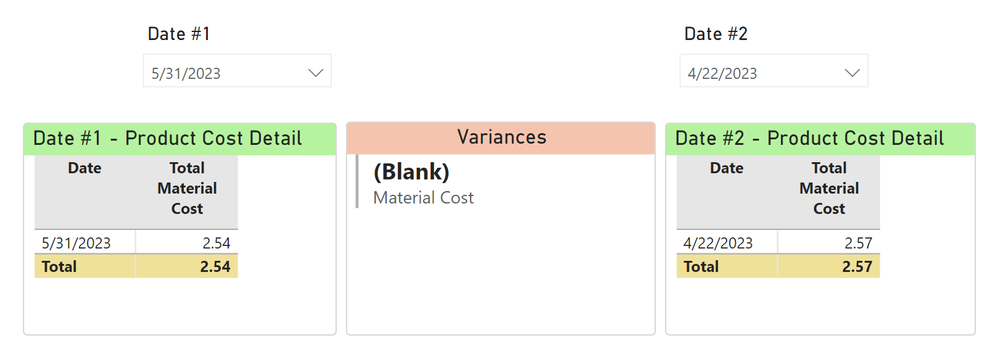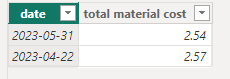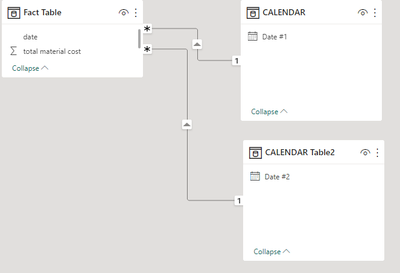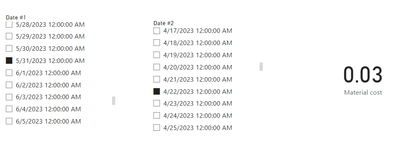cancel
Showing results for
Did you mean:Helper I

## How to get variance based on two date slicers

Hello all,

I have a report where I need to show a variance based on selections in two date slicers.  See screenshot.  I have a single fact table connected to a date table.  That single date table is used to populate both slicers, and those slicers interact visually with each table based on the selection.  For the "Variance" measure, I've tried all manner of things based on search results, but none seem to pinpoint my exact (and hopefully simple) need here.  I won't muddy the waters by showing what I've already tried.

In the sample below, the expected result should be 0.03.  Can anyone point me in the right direction here?  Thanks in advance!1 ACCEPTED SOLUTIONCommunity Support

Hi @wbhite ,

According to your description, here are my steps you can follow as a solution.

(1) This is my test data.

Fact table:Calendar table:

``CALENDAR = CALENDAR(DATE(2023,1,1),DATE(2023,12,31))``

(2) We can create a table and a measure.

``CALENDAR Table2 = VALUES('CALENDAR'[Date #1])````````Material cost =
var _a=CALCULATE(MAX('Fact Table'[total material cost]),FILTER(ALL('Fact Table'),'Fact Table'[date]=SELECTEDVALUE('CALENDAR'[Date #1])))
var _b=CALCULATE(MAX('Fact Table'[total material cost]),FILTER(ALL('Fact Table'),'Fact Table'[date]=SELECTEDVALUE('CALENDAR Table2'[Date #2])))
return _b-_a``````

(3) Then the result is as follows.Best Regards,

Neeko Tang

If this post  helps, then please consider Accept it as the solution  to help the other members find it more quickly.

3 REPLIES 3Super User

Hi,

I cannot understand your requirement.  Why in the first place do you have 2 date slicers?  Do you want to compare the cost of 2 products between a week of each other?

Regards,
Ashish Mathur
http://www.ashishmathur.comCommunity Support

Hi @wbhite ,

According to your description, here are my steps you can follow as a solution.

(1) This is my test data.

Fact table:Calendar table:

``CALENDAR = CALENDAR(DATE(2023,1,1),DATE(2023,12,31))``

(2) We can create a table and a measure.

``CALENDAR Table2 = VALUES('CALENDAR'[Date #1])````````Material cost =
var _a=CALCULATE(MAX('Fact Table'[total material cost]),FILTER(ALL('Fact Table'),'Fact Table'[date]=SELECTEDVALUE('CALENDAR'[Date #1])))
var _b=CALCULATE(MAX('Fact Table'[total material cost]),FILTER(ALL('Fact Table'),'Fact Table'[date]=SELECTEDVALUE('CALENDAR Table2'[Date #2])))
return _b-_a``````

(3) Then the result is as follows.Best Regards,

Neeko Tang

If this post  helps, then please consider Accept it as the solution  to help the other members find it more quickly.Helper I

This is great, thanks so much!